# Introduction..

The RNN based encoder and decoder models are proved to be very powerful neural architecture which provides a practical solution to many sequence to sequence predictions problems like machine translation, question answering model and text summarization.
The encoder in the model is tasked with building a contextual representation if input sequence. The decoder , which uses the context to generate the output sequence. In RNN context we described in the last blog, the context vector is essentially the last hidden state of the last time step“ hn” in the chain of input sequence. This final hidden state/ context vector must represent absolutely everything about the meaning of the input sequence and final hidden state/ context vector is the only thing that decoder knows about the input text.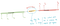Illustration of Bottleneck issue, generated by author

So it clearly seems that the final hidden state is acting some sort of bottleneck. As the input sequence length of the input increases, encoding the entire information in that context vector becomes infeasible. The attention mechanism solves this bottleneck problem in a way that decoder knows not only the final hidden state but hidden states of all encoder time steps. In this blog, we will discuss about the attention mechanism and how it solves for bottleneck issue.

# Attention Mechanism..

In vanilla encoder-decoder model, the context is a single vector which is a function of the last hidden state of the encoder RNN. Attention mechanism proposes usage the all hidden states from the encoder network since each hidden state carries an information that can influence the the decoder output at any timestep. Attention mechanism is based on the concept that instead of using one last hidden state, we use hidden states at all time-steps of input sequence for better modelling of long-distance relationships.

To achieve this, the idea of attention is to create a context vector “Ci” which is weighted sum of all the encoder hidden states. So the context vector is not static anymore, we will have different context vectors at each time step of decoding. The context vector “Ci” is generated anew at each decoding step i by applying weighted sum of all the hidden states of encoder.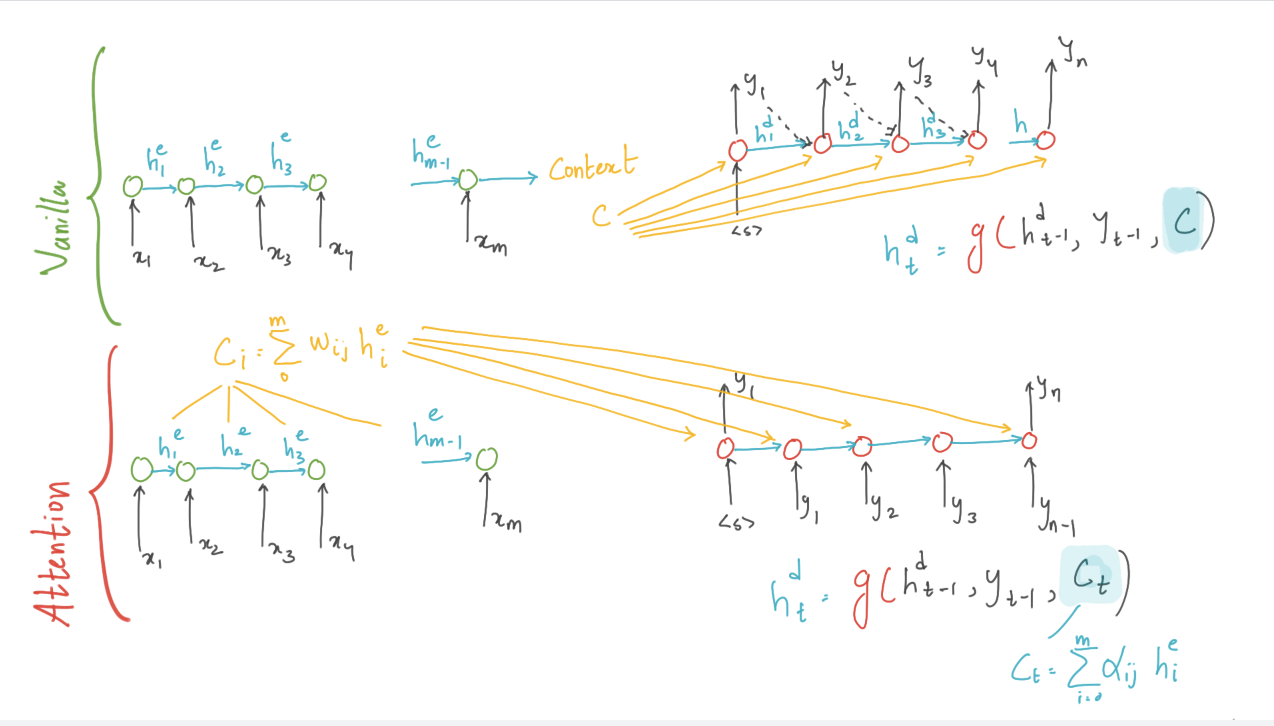illustration of vanilla and attention encoder-decoder model, generated by author

## Weights of Attention

The weights are used to focus on a particular part of input sequence that is relevant for the token currently being produced by the decoder.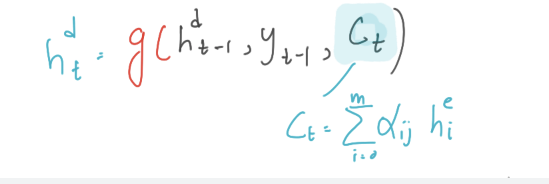hidden state calculation at decoding step t, generated by author

So at each time step of decoding, we compute a context “ct” which is made available for computing the decoding hidden state at time step t. The first step in computing ct is to compute the weights/relevance on each encoder state. The weight can be seen as a score of relevance that each encoder state hj that has while computing the decoder at time step t.

## Dot-Product Attention

We can compute the relevance or weights by computing the similarity between decoder hidden state to the encoder hidden state.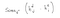Score equation, generated by author

The output from this dot product gives us the degree of similarity, this score across all the hidden states will give us the relevance of every encoder state to the current step of decoder. We will have to normalise the scores to get the weights.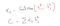Dynamic context vector equation, generated by author

Now we finally arrived at a method to calculate the dynamic context vectors that takes into account from the entire hidden states while encoding the input sequence. Dot product attention helps us understand the essence of attention mechanism itself. But its also possible to create more sophisticated scoring function. We will discuss them briefly in coming sections

## Parameterised Attention

By parametrising the scoring functions instead of using simple dot product, we can derive more powerful scoring functions. where the weights Ws are learned in the training process.

# Note:

Soft vs. Hard Attention: The techniques discussed so far are soft attention mechanism where the decoder picks the weighted average over all the hidden states in encoder network. The only difference between soft attention and hard attention is that in hard attention it picks one of the encoder states. Hard attention is not differentiable and hence cannot be used in standard back-propagation methods.

RL | ML | ALGO TRADING | TRANSPORTATION | GAME THEORY